# What does Poissons equation really mean

The Poisson's equation (after Siméon Denis Poisson) describes a boundary value problem in which the derivatives of a vector field on the surface of a volume are given. This is used, for example, in electrostatics (Gauss law). One can also determine the gravitational potential of a given mass distribution.

The Poisson equation is a partial differential equation of the second order: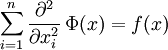or shorter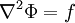or

ΔΦ = f

i.e. in the Poisson's equation the Laplace operator Δ applied to a function Φ becomes equal f set.

The homogeneous form of Poisson's equation is the Laplace equation.

### Electrostatics

Since the electric field is a conservative field, it can be over the gradient of a potential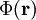be expressed with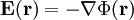.

Using another Nabla operator results in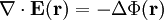.

However, according to the first Maxwell equation, the following also applies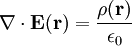,

in which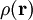the charge density and ε0 which are permittivity.

It follows that for the Poisson equation of the electric field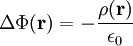### Gravity

The gravitational acceleration results from the law of gravitation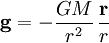.

The flow through the surface of any volume is then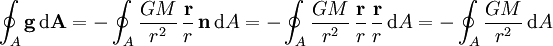,

in which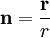is the normal vector. The following applies in spherical coordinates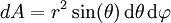,

From which follows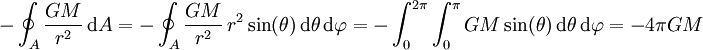From one by one mass densityThe mass distribution described results in the total mass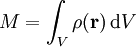.

So it follows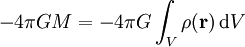.

With Gauss's theorem, however, we also get for the integral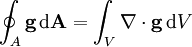,

and thus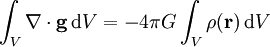.

Since the shape of the volume is arbitrary, the integrands must be equal, so that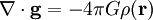is. The gravity represents a conservative force field, so the relationship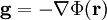applies. This gives the Poisson equation for gravity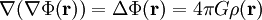,

the minus sign disappears.

Category: Electrostatics